# Mathematics Paper 2 Questions And Answers - Form 4 Term 2 Opener 2021

INSTRUCTIONS TO CANDIDATES:

• This paper consists of TWO sections: Section I and Section II.
• Answer ALL the questions in section I and only five from Section II
• All answers and working must be written on the question paper in the spaces provided below each question.
• Show all the steps in your calculations, giving your answers at each stage in the spaces below each question.
• Marks may be given for correct working even if the answer is wrong.
• Non-programmable silent electronic calculators and KNEC Mathematical tables may be used except where stated otherwise.
1. Evaluate without using Mathematical tables or a calculator. (3mks)
2log 5 − ½log 6 + 2log 40
2. Solve for x given that the following is a singular matrix (2mks)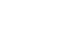3. Make d the subject of the formula. (3mks)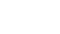4. Simplify     3     +   leaving your answer in the form a + b√c, where a, b and c are rational numbers.
√(7−2)    √7                (3mks)
5. Calculate the percentage error in the volume of a cone whose radius is 9.0cm and slant length 15.0cm. (3mks)
6. A quantity A is partly constant and partly varies inversely as a quantity B. Given that A = −10 when B= 2.5 and A = 10 when B = 1.25, find the value of A when B = 1.5. (4mks)
7. The table below shows corresponding values of x and y for a certain curve.
 y 1 1.2 1.4 1.6 1.8 2 2.2 x 6.5 6.2 5.2 4.3 4 2
Using 3 strips and mid-ordinate rule, estimate the area between the curve x axis, the line x = 1 and x = 2.2. (2mks)
8. 14 people can build 10 huts in 30 days. Find the number of people working at the same rate that will build 18 similar huts in 27 days. (3mks)
9. The coordinates of two airports M and N are (60°N, 35°W) and (60°N, 15°E) respectively. Calculate;
1. The longitude difference. (1mk)
2. the shortest time an aeroplane whose speed is 250 knots will take to fly from M to N along a circle of latitude. (2mks)
10.
1. Expand (x − 0.2)5 in ascending powers of x. (2mks)
2. Use your expansion up to the fourth term to evaluate 9.85. (2mks)
11. The figure below is a cuboid ABCDEFGH. AB = 12cm, BC = 5cm and CF = 6.5cm.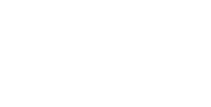1. State the projection of AF on the plane ABCD. (1mk)
2. Calculate the angle between AF and the plane ABCD correct to 2 decimal planes. (3mks)
12. Show that sinx( cosx + 1) =sinx + tanx .
cosx
13. The mid-point of AB is (1,−1.5, 2) and the position vector of a point A is −1 +j. Find the magnitude of

AB where O is the origin. (3mks)
14. Draw a line of best fit for the graph of y against x using the values in the table below. Hence determine the equation connecting y and x.
 x 0.4 1 1.4 2 2.5 y 0.5 1 1.2 1.5 2
15. A coffee dealer mixes two brands of coffee, x and y to obtain 40kg of the mixture worth Ksh. 2,600. If brand x is valued at Ksh. 70 per kg and brand y is valued at Ksh. 55 per kg. Calculate the ratio in its simplest form in which brands x and y are mixed. (4mks)
16. The figure below shows a circle centre O. AB and PQ are chords intersecting externally at a point C. AB = 9cm, PQ= 5cm and QC = 4cm. Find the length of BC. (3mks)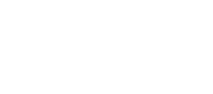SECTION II (50 MARKS)

Answer only five questions in this section

1.
1. Salome invested Ksh. 250,000 for 2 ½ years in an account which paid 16% compound interest p.a. The interest is compounded quarterly. At the end of 2 ½ years she withdrew all the amount and spent it to the nearest thousands to buy four similar motor cycles. She earned an average of Ksh. 10,000 from each motorcycle per month.
1. the amount she withdrew at the end of 2 ½ years. (2mks)
2. the cost of each motorcycle. (2mks)
3. the total earnings from the motorcycles for 3 years. (2mks)
2. She decided to sell the motorcycles after depreciating at an average rate of 20% p.a for the 3 years.
Find:-
1. the new value of each motorcycle after depreciation. (2mks)
2. the profit earned from her initial investment to the nearest shilling. (2mks)
2. The table below shows the distribution of ages in years of 50 adults who attended a clinic:-
 Age 21-30 31-40 41-50 51-60 61-70 71-80 Frequency 15 11 17 4 2 1

1. State the medium class (1mk)
2. Using a working mean of 45.5, calculate:-
1. the mean age (3mks)
2. the standard deviation (3mks)
3. Calculate the 6th docile. (3mks)
3. An arithmetic progression (AP) has the first term a and the common difference d.
1. Write down the third, ninth and twenty fifth terms of the AP in terms of a and d. (1mk)
2. The AP above is increasing and the third, ninth and twenty fifth terms form the first three consecutive terms of a Geometric Progression (G.P) The sum of the seventh and twice the sixth terms of the AP is 78. Calculate:-
1. the first term and common difference of the AP. (5mks)
2. the sum of the first nine terms of the AP. (2mks)
3. The difference between the fourth and the seventh terms of an increasing AP.    (2mks)
4. The probability that three candidates; Anthony, Beatrice and Caleb will pass an examination are ¾, 2/3 and 4/5 respectfully. Find the probability that:
1. all the three candidates will pass (2mks)
2. all the three candidates will not pass. (2mks)
3. only one of them will pass (2mks)
4. only two of them will pass. (2mks)
5. at most two of them will pass. (2mks)
5.
1. Complete the table below for the function y = (3 − x) (x +1)
 x −3 −2 −1 0 1 2 3 4 x+1 −2 −1 1 3 4 3−x 6 5 4 2 1 −1 y −12 −5 3 4 0 −5
(2mks)
2. Use the values in the table to draw the graph of y = (3 − x) (x +1). Use the following scale.
Horizontal axis 2cm for 1 unit
Vertical axis 1cm for 1 unit. (3mks)
3. Use your graph in part (b) above to solve the following quadratic equations
1. −x2 + 2x + 3 = 0 (2mks)
2. −x2 + x + 6 = 0   (3mks)
6. Use a ruler and a pair of compasses only all constructions in this question.
1. Construct the rectangle ABCD such that AB = 7.2cm and BC = 5.6cm. (3mks)
2. Constructs on the same diagram the locus L1 of points equidistant from A and B to meet with another locus L2 of points equidistant from AB and BC at M. measure the acute angle formed at M by L1 and L2. (3mks)
3. Construct on the same diagram the locus of point K inside the rectangle such that K is less than 3.5cm from point M. Given that point K is nearer to B than A and also nearer to BA than BC, shade the possible region where K lies. Hence calculate the area of this region. Correct to one decimal place. (4mks)
7. The diagram below, not drawn to scale shows part of the curve y = x2 + 5 and the line y = 8 − 2x.
The line intersects the curve at points C and D. Lines AC and BD are parallel to the y-axis.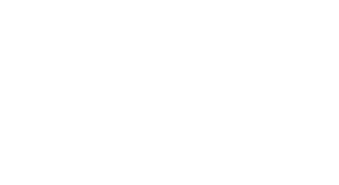1. Determine the coordinates of C and D. (4mks)
2. Use integration to calculate the area bounded by the curve and the x-axis between the points C and D. (3mks)
3. Calculate the area enclosed by the lines CD, CA, BD and the x-axis. (3mks)
4. Hence determine the area of the shaded region. (1mk)
8. A tailoring business makes two types of garments A and B. Garment A requires 3 metres of material while garment B requires 2 ½ metres of material. The business uses not more than 600 metres of material daily in making both garments. It must make not more than 100 garments of type A and nor less than 80 of type B each day.
1. Write down three inequalities from this information other than x ≥ 0and x ≥ y, where x is the number of garments of type A and y the number of garments of type B. (3mks)
2. Graph these inequalities. (3mks)
3. If the business makes a profit of sh 80 on garment A and a profit of sh. 60 on garment B, how many garments of each type must it make in order to maximize the profit and what is the total profit? (4mks)## MARKING SCHEME

1. log 52− log16½ log 402
log 25 − log 4 + log1600
=log( 25 × 1600)
4
=log 10,000
=4
2. 2x − (x−3) = 0
2x − x + 3 = 0
x =−3
3. a4 = 1 + d2 + b
b2       3
a4 −  b1 + d2
3       b2
b2(a4 − b/3) = 1 + d2
b2(a4 − b/3) − 1 = d2
d = √(b2(a4 − b/3)−1)
4. = 3√7 + √7 − 2
√7 (√7 − 2)
= 4√(7−2)
7−2√7
4√(7−2) ×  7+ 2√7
7−2√7       7+ 2√7
4√(7+(7+2√7)−2(7+ 2√7)
21
= 28√7+56 − 14 − 4√7
21
= 42+24√7
21
= 2 + 8/7√7
5. height = √(152 − 92) = 12.0cm
v = 1/3 × π × 9.0 × 9.0 × 12.0
% error =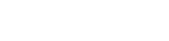=1.528%
6. A = k + m/B
−10 =  k + m/2.5       (i)
−25 + 2.5k +m
10 = k + m/12.5      (ii)
12.5 = 1.25k + m
−25 = 2.5k +m
12.5 = 1.25k + m
−37.5 =1.25k
k = −30
12.5 = 1.25 (−30) + m
m=50
A =−30 + 50/B
= −30 + 50/1.5
= 31/3
7. Area = h (y1+y2………………..)
= 0.4 (6.2+4.3+2.6
= 5.24
8. = 14 × 18/10 × 30/27
=28 people
9.
1. 35+15 = 50°
2.  Time  = 60 ×50 × cos60
250
= 6hrs
10.
1. = x5 + 5x4 (−0.2) + 10x3 (−0.2)2 +10x2 (−0.2)2 + 5x(−0.2)4
= x5 − x4 + 0.4x3 − 0.08x2 +0.008x − 0.00032
2. = 105 − 104 + 0.4(10)3 − 0.08(10)2
= 90392
11.
1. AC
2. AC = √(125 + 52) =13cm
tan θ = 6.5
13
θ = 26.57°
12. sinx cosx + sinx
cosx
sinx cosx + sinx
cosx         cosx
sinx + tanx
13. A(−1,1,0)           B(x,y,z)
→ −1 + x =1, 1 + y =−1.5, 0 + z =2
2              2                  2
x=3, y=−4, z=4
B(3, −4.4)
/OB/ = √(32 +(−4)2 +42)
=√41
=6.403 units
14.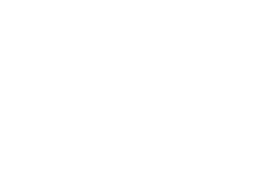y = 0.7x + 0.2
15. 2600 = 65
40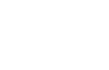= 2:3
16. x(x+9) =36
x2 + 9x − 36 =0
(x+12) (x−3) =0
x =−12, x=3
BC = 3cm
17.
1.
1. 250,000( 1+ 4/100)10
=sh 370061.0712
2. 370,000
4
=sh. 92,500
3. 10000 × 4 × 36
= sh 1,440,000
2.
1. 92500 ( 1 − 20/100)3
=sh 47,360
2. Profit = (61+ 1440000+189440)− 250 000
=1379 501
18.
 Class x tx−45.5 f ft ft2 cf 21-30  31-40 41-50 51-60 61-70 71-80 25.5  35.5 45.5 55.5 65.5 75.5 −20 −10    0   10   20   30 15  11 17 4 2 1 −300  −110    0   40   40   30 6000  1100   0 400 800 900 15  26 43 47 49 50 50 −300 9200

1. 31-40
2.
1. Mean =  −300 + 45.5
50
=39.5yrs
2. s.d =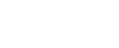=12.17
3. 6th = 40.5 + (30 − 26)10
17
=42.85
19.
1. a+2d, a+8d, a+24d
2.
1. a+8d  = a+24d           I
a+2d     a+8d
a+6d+2(a+5d)= 78    II

16d = 10a         I
3a +16d =78    II

3a +10a =78
13a =78
a=6

16d = 10 × 6
d = 3.75
2. S49/2 (2 × 6 +( 9−1)3.75)
=189
3. →(6+6(3.75)) − (6+3(3.75))
=11.25
20. P(A) = ×    P(A1) =¼
P(B) = 2/3   P(B1) = 1/3
P(C) = 4/5     P(C1) = 1/5
1. P(ABC) = ¾ × 2/3 × 4/5
= 2/5
2. P(A1B1C1) = ¼ × 1/3 × 1/5
= 1/60
3. →P(A1B1C1 or (A1BC1   A1B1C)
=(¾ × 1/3 × 1/5) + (¼ × 2/3 × 1/5) + (¼ × 2/3 × 4/5)
9/60
4. →P(ABC1 or AB1C or  A1BC)
(¾ × 2/3 × 1/5) + (¾ × 1/3 × 1/5) + (¼ × 2/3 × 4/5)
=26/60
5. → 1 − P(ABC)
= 1 − 2/5
=3/5
21.
1.
 x −3 −2 −1 0 1 2 3 4 x+1 −2 −1 0 1 2 3 4 5 3−x 6 5 4 3 2 1 0 −1 y −12 −5 0 3 4 3 0 −5
2.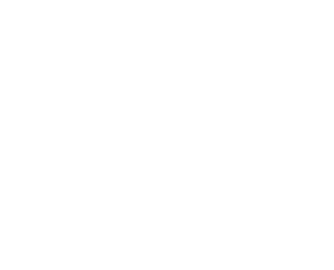3.
1. (3−x) (x+1) = 0
−x2+2x+3=0
x=3,−1
2. −x2 +2x +3 = y
−x2+x+6=0
x−3= y
x=−2, 3
22.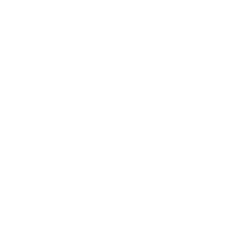44/360 × 22/7 × 3.5 × 3.5
= 4.7cm2
23.
1. x2 + 5 = 8 − 2x
x2 + 2x − 3 = 0
(x +3) (x−1) =0
x = −3 or 1
When x=−3,  y=14
When x =1, y = 6
coordinates
C(−3,14) and D(1,6)
2.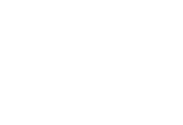=321/square units
3. Area under line y=8 − 2x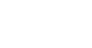=(8(1) −12) −(8(−3)−(−3)2)
=40
4. Shaded area =40 − 321/3
=72/3
24.
1. 3x + 2.5y ≤ 600
x ≤ 100
y ≥ 80
2.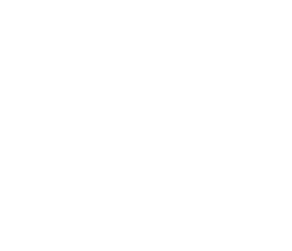3. Solution (100, 120)
100type A and 120 type B garments
Profit=80 x 100 + 60 x 120
=sh. 15, 200

• ✔ To read offline at any time.
• ✔ To Print at your convenience
• ✔ Share Easily with Friends / Students